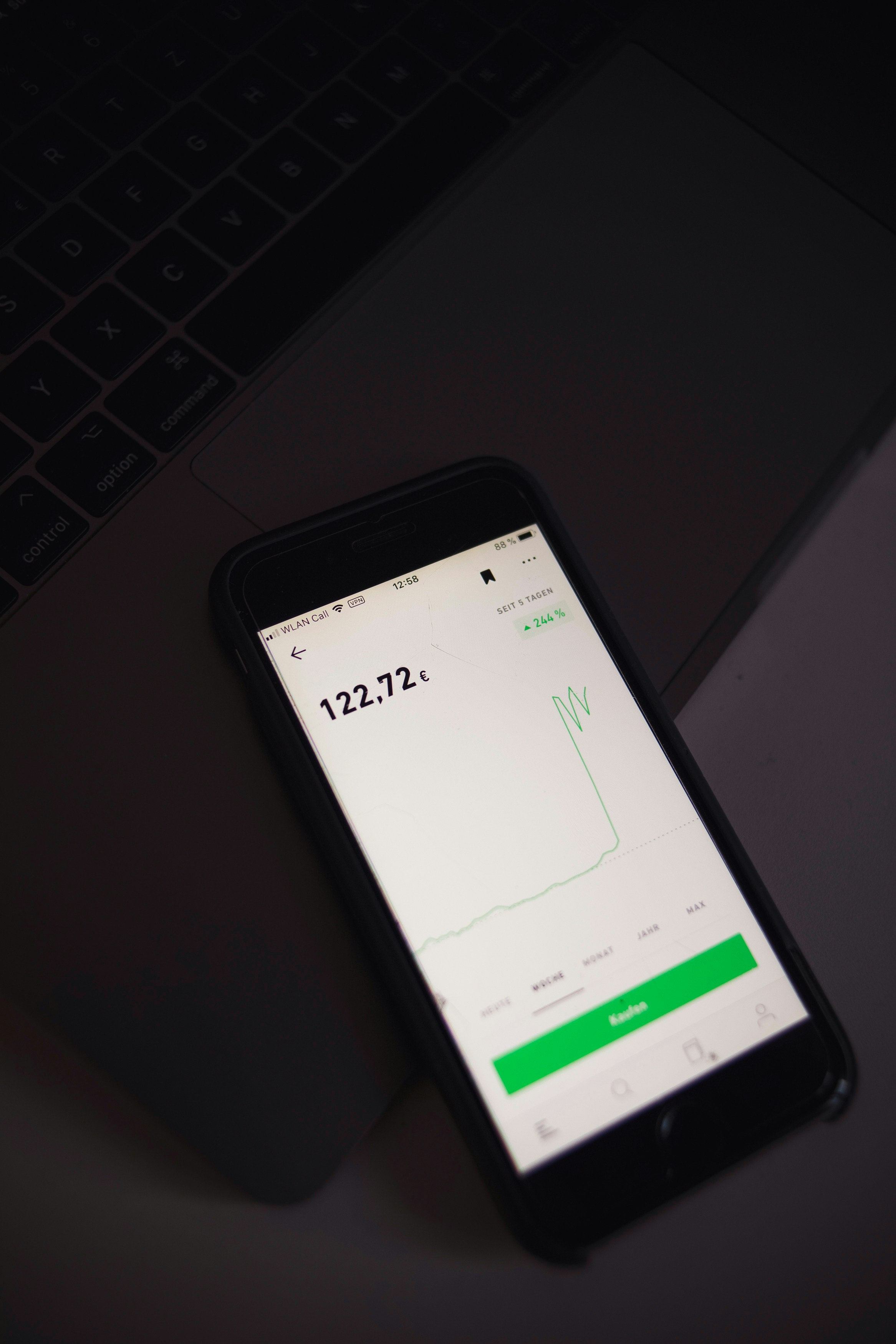# How to Calculate the Dividend Payout RatioInvesting News

A company’s dividend payout ratio gives investors an idea of how much money it returns to its shareholders compared to how much it keeps on hand to reinvest in growth, pay off debt, or add to cash reserves.

This ratio is easily calculated using the figures found at the bottom of a company’s income statement. It differs from the dividend yield, which compares the dividend payment to the company’s current stock price.

### Key Takeaways

• The dividend payout ratio is a way to find out how much money in dividends is paid out.
• This calculation allows companies to find out how much money is left over (after the dividends are paid) to use for paying down debts or reinvesting.
• You calculate this ratio using a company’s income statement.
• A dividend payout ratio is different than a retention ratio or the dividend yield
• Companies use this ratio, not individuals.

## Calculating the Dividend Payout Ratio

The dividend payout ratio can be calculated as the yearly dividend per share divided by the earnings per share (EPS), or equivalently, or divided by net income dividend payout ratio on a per share basis. In this case, the formula used is dividends per share divided by earnings per share (EPS). EPS represents net income minus preferred stock dividends divided by the average number of outstanding shares over a given time period. One other variation preferred by some analysts uses the diluted net income per share that additionally factors in options on the company’s stock.

## Where to Find Dividend Payout Ratio Numbers

The figures for net income, EPS, and diluted EPS are all found at the bottom of a company’s income statement. For the amount of dividends paid, look at the company’s dividend announcement or its balance sheet, which shows outstanding shares and retained earnings.

A growth investor interested in a company’s expansion prospects is more likely to look at the retention ratio, while an income investor more focused on analyzing dividends tends to use the dividend payout ratio.

## Dividend Payout Ratio vs. Retention Ratio

The dividend payout ratio is the opposite of the retention ratio which shows the percentage of net income retained by a company after dividend payments. The payout ratio indicates the percentage of total net income paid out in the form of dividends.

Calculating the retention ratio is simple, by subtracting the dividend payout ratio from the number one. The two ratios are essentially two sides of the same coin, providing different perspectives for analysis.

For example, a company pays out \$100 million in dividends per year and made \$300 million in net income the same year. In this case, the dividend payout ratio is 33% (\$100 million ÷ \$300 million). Thus, the company pays out 33% of its earnings via dividends. Meanwhile, its retention ratio is 66%, or 1 minus the dividend payout ratio (1 – 33%). Thus, the company retains 66% of its net income for reinvesting.

## Dividend Payout Ratio vs. Dividend Yield

While many investors are focused on the dividend yield, a high yield might not necessarily be a good thing. If a company is paying out the majority, or over 100%, of its earnings via dividends, then that dividend yield might not be sustainable.

For example, a company offers an 8% dividend yield, paying out \$4 per share in dividends, but it generates just \$3 per share in earnings. That means the company pays out 133% of its earnings via dividends, which is unsustainable over the long term and may lead to a dividend cut.

## What Is a Dividend?

Dividends are earnings on stock paid on a regular basis to investors who are stockholders.

## What Is a Good Dividend Payout Ratio?

It may vary depending on the situation but overall a good payout ratio on dividends is considered to be anywhere from 30% to 50%.

## How Can I Calculate a Dividend Payout Ratio?

The dividend payout ratio can be calculated by taking the yearly dividend per share and dividing it by the earnings per share or you can use the dividends divided by net income.